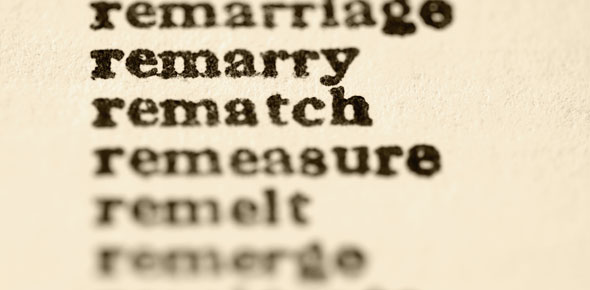# Data Vocabulary Quiz 2

20 Questions | Attempts: 172SettingsStatistics/Analyzing Data Vocabulary Test�

• 1.
The horizontal number line on a graph
• A.

Title

• B.

X - axis

• C.

Y - axis

• D.

Interval

• 2.
The _____ of a set of data is the number or numbrs that occur most often.
• A.

Range

• B.

Mean

• C.

Median

• D.

Mode

• 3.
A _____ uses bars to represent the frequency of data that have been organized in intervals.
• A.

Bar graph

• B.

Histogram

• C.

Line plot

• D.

Stem-and-leaf plot

• 4.
Numbers called _____ can be used to describe the center of data.
• A.

Measures of central tendency

• B.

Data

• C.

Intervals

• D.

Scale

• 5.
The vertical number line on a graph
• A.

Title

• B.

Label

• C.

X-axis

• D.

Y-axis

• 6.
A type of graph that looks like a number line with each piece of data displayed as an x above the number line.
• A.

Line plot

• B.

Scatter plot

• C.

Stem-and-leaf plot

• D.

None of these

• 7.
A graphic form using bars that do not touch to make comparisons of statistics.
• A.

Line plot

• B.

Stem-and-leaf plot

• C.

Histogram

• D.

Bar graph

• 8.
A graph that has a column for ones place value and ten place value
• A.

Line plot

• B.

Stem-and-leaf plot

• C.

Scatter plot

• D.

Bar graph

• 9.
Groups of numbers - This information goes on the x-axis of a histogram.
• A.

Interval

• B.

Frequency

• C.

Label

• D.

Title

• 10.
Another word for average - The sum of the data divided by the number of items in the data set.
• A.

Range

• B.

Mean

• C.

Median

• D.

Mode

• 11.
The middle number in a set of data that has been ordered least to greatest
• A.

Range

• B.

Mean

• C.

Median

• D.

Mode

• 12.
The difference between the greatest and least numbers in a data set.
• A.

Range

• B.

Mean

• C.

Median

• D.

Mode

• 13.
A table for organizing a set of data that shows the number of pieces of data that fall within given intervals or categories.
• A.

Title

• B.

Range

• C.

Frequency table

• D.

Stem-and-leaf plot

• 14.
A piece of data that is quite separated from the rest of the data.
• A.

Outlier

• B.

Mean

• C.

Median

• D.

Mode

• 15.
Data that are grouped closely together.
• A.

Outlier

• B.

Cluster

• C.

Gap

• D.

Range

• 16.
The upper median of a set of data
• A.

Lower extreme

• B.

Lower quartile

• C.

Upper quartile

• D.

Upper extreme

• 17.
The lower median of a set of data
• A.

Lower extreme

• B.

Lower quartile

• C.

Upper quartile

• D.

Upper extreme

• 18.
The least number in a set of data
• A.

Lower extreme

• B.

Lower quartile

• C.

Upper quartile

• D.

Upper extreme

• 19.
The greatest number in a set of data
• A.

Lower extreme

• B.

Lower quartile

• C.

Upper quartile

• D.

Upper extreme

• 20.
The difference between the upper quartile and the lower quartile is known as
• A.

Interquartile range

• B.

Lower extreme

• C.

Upper extreme

• D.

Median

## Related TopicsBack to top
×

Wait!
Here's an interesting quiz for you.